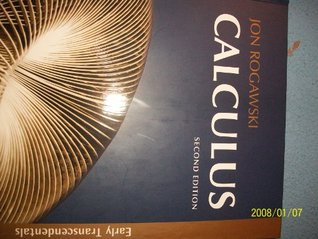Home » Calculus (Early Transcendentals, 2nd edition) by Jon Rogawski# Calculus (Early Transcendentals, 2nd edition)

## Jon Rogawski

Published
ISBN :
Hardcover
1050 pages
Book Rating:Enter the sum

 About the Book Very good used condition. Calculus 2nd Edition. Good guide to learning calculus from easier concepts to the more complicated ones.Chapters:1. Precalculus Review2. Limits3. Differentiation4. Applications of the Derivative5. The Integral6.MoreVery good used condition. Calculus 2nd Edition. Good guide to learning calculus from easier concepts to the more complicated ones.Chapters:1. Precalculus Review2. Limits3. Differentiation4. Applications of the Derivative5. The Integral6. Applications of the Integral7. Exponential Functions8. Techniques of Integration9. Further Applications of the Integral and Taylor Polynomials10. Introduction to Differential Equations11. Infinite Series12. Parametric Equations, Polar Coordinates, and Conic Sections13. Vector Geometry14. Calculus of Vector-Valued Functions15. Differentiation in Several Variables16. Multiple Integration17. Line and Surface Integrals18. Fundamental Theorems of Vector Analysis